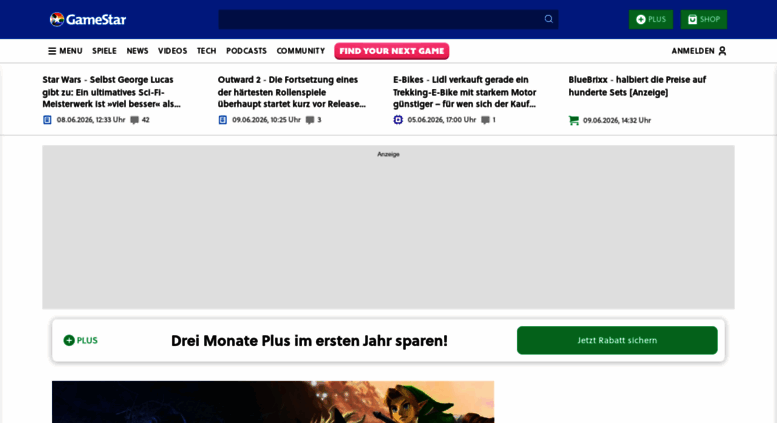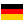# gamestar.de: GameStar – Das Nr. 1 Magazin für PC-Spieler

GameStar.de versorgt euch mit Tests, Previews, Videos, Reportagen und News zu PC-Spielen und Hardware. Wir liefern Spielejournalismus, dem ihr vertrauen könnt.

• 55/100

Normal result
• 6

Successful tests
• 2

Failed tests
• First response

299 ms

4.5 sec

• Page rendered

571 ms

5.4 sec

Click here to check amazing Game Star content for Germany. Otherwise, check out these important facts you probably never knew about gamestar.de

We analyzed Gamestar.de page load time and found that the first response time was 299 ms and then it took 5.1 sec to load all DOM resources and completely render a web page. This is a poor result, as 70% of websites can load faster.

## Page optimization

•  HTML 271.2 kB Images 1.6 MB Javascripts 886.2 kB CSS 866.3 kB

In fact, the total size of Gamestar.de main page is 3.6 MB. This result falls beyond the top 1M of websites and identifies a large and not optimized web page that may take ages to load. 70% of websites need less resources to load. Images take 1.6 MB which makes up the majority of the site volume.

• Original 271.2 kB
• After minification 270.3 kB
• After compression 59.6 kB

HTML optimization

HTML content can be minified and compressed by a website’s server. The most efficient way is to compress content using GZIP which reduces data amount travelling through the network between server and browser. HTML code on this page is well minified. It is highly recommended that content of this web page should be compressed using GZIP, as it can save up to 211.6 kB or 78% of the original size.

• Original 1.6 MB
• After optimization 1.4 MB

Image optimization

Image size optimization can help to speed up a website loading time. The chart above shows the difference between the size before and after optimization. Game Star images are well optimized though.

• Original 886.2 kB
• After minification 835.7 kB
• After compression 279.6 kB

JavaScript optimization

It’s better to minify JavaScript in order to improve website performance. The diagram shows the current total size of all JavaScript files against the prospective JavaScript size after its minification and compression. It is highly recommended that all JavaScript files should be compressed and minified as it can save up to 606.6 kB or 68% of the original size.

• Original 866.3 kB
• After minification 680.0 kB
• After compression 120.6 kB

CSS optimization

CSS files minification is very important to reduce a web page rendering time. The faster CSS files can load, the earlier a page can be rendered. Gamestar.de needs all CSS files to be minified and compressed as it can save up to 745.7 kB or 86% of the original size.

### Network requests diagram

• gamestar.de
• www.gamestar.de
• royalslider.css
• rs-default.css
• combine.css
• jquery.min.js
• iam.js
• css
• combine.js
• jquery.autocomplete.js
• suggest.js
• product.js
• page.js
• home.js
• countdown.js
• lightbox.js
• dlightbox.js
• bid
• analytics.js
• 72x104.jpg
• original.jpg
• 298x168.jpg
• 298x168.jpg
• 298x168.jpg
• 298x168.jpg
• 285x.jpg
• 68x51.jpg
• 298x168.jpg
• bgNaviPlus.png
• b144x81.jpg
• 780585960.jpg
• 279x157.jpg
• logo3.png
• btnClose.png
• btnZoomImage.png
• original.jpg
• 68x51.jpg
• 112x63.jpg
• 128x72.jpg
• x198.jpg
• x198.jpg
• x198.jpg
• 72x104.jpg
• 298x168.jpg
• 128x72.jpg
• 128x72.jpg
• 112x63.jpg
• 279x157.jpg
• 146x83.jpg
• b144x81.jpg
• iab.cfm
• b144x81.jpg
• 279x157.jpg
• 279x157.jpg
• 279x157.jpg
• 128x72.jpg
• x198.jpg
• x198.jpg
• 298x168.jpg
• 298x168.jpg
• 112x63.jpg
• 287x.jpg
• 146x83.jpg
• 112x63.jpg
• b144x81.jpg
• 112x63.jpg
• b144x81.jpg
• 128x72.jpg
• 744602483.jpg
• 747815727.jpg
• 698734610.jpg
• 697064051.jpg
• ec.js
• collect
• collect
• tx.io
• tx.io
• gamestarde.js
• globalV6.js
• rc
• c.ount
• gw.js
• pql
• pixel
• pixel
• default.js
• oba_icon.png
• 8106,82935,8110,157470,8108,82934,24808
• p.min.js
• data
• c.ount
• www.gamestar.de;s_pid=1;fv=0;sx=1680;sy=1050;zz=1x1;site=DE_Gamestar.de;zone=home;idf=gamestar;c1=0;c2=0;c3=0;c4=1;c5=1;c6=0;c7=0;c8=0;c9=0;c10=0;c11=1;c12=0;c13=0;c14=0;c15=0;c16=1;c17=0;c19=0;c20=0;d1=1;d3=5;d4=0;d8=1;d9=3;d10=1;d12=1;d15=3;d16=1;d17=1;d20=1;d21=0;d22=-1;i55=0;i3=1;i1=1;i13=1;i21=1;i28=1;i42=1;i46=1;i53=1;n3=1;n5=1;n8=1;n9=0;f1=1;f2=1;f3=1;xx=pl;xx=fa;xx=pb;xx=pd;xx=bb;xx=wp;xx=fp;xx=hp;xx=sb;xx=pu1;xx=fb2;xx=rt1;xx=sc1;!c=ga;tile=1;ord=9684122893
• 745073516.js
• rs.js
• rpc.flow
• vce_st.js
• tab.cfm
• gtm.js
• btnArrowDown2.png
• suche_01.png
• btnSearch.png
• bgNaviMain.png
• p2
• www.gamestar.de;s_pid=1;fv=0;sx=1680;sy=1050;zz=468x60;site=DE_Gamestar.de;zone=home;idf=gamestar;c1=0;c2=0;c3=0;c4=1;c5=1;c6=0;c7=0;c8=0;c9=0;c10=0;c11=1;c12=0;c13=0;c14=0;c15=0;c16=1;c17=0;c19=0;c20=0;d1=1;d3=5;d4=0;d8=1;d9=3;d10=1;d12=1;d15=3;d16=1;d17=1;d20=1;d21=0;d22=-1;i55=0;i3=1;i1=1;i13=1;i21=1;i28=1;i42=1;i46=1;i53=1;n3=1;n5=1;n8=1;n9=0;f1=1;f2=1;f3=1;xx=pl;xx=fa;xx=pb;xx=pd;xx=bb;xx=wp;xx=fp;xx=hp;xx=sb;xx=pu1;xx=fb2;xx=rt1;xx=sc1;!c=ga;tile=2;ord=9684122893
• c.ount
• p
• d4d3d3354b.js
• pixel
• generic
• generic
• zrt_lookup.html
• match
• match
• match
• match
• match
• som-script.php
• p
• rpc.flow
• p
• osd.js
• c.ount
• www.gamestar.de;s_pid=1;fv=0;sx=1680;sy=1050;zz=160x600;site=DE_Gamestar.de;zone=home;idf=gamestar;c1=0;c2=0;c3=0;c4=1;c5=1;c6=0;c7=0;c8=0;c9=0;c10=0;c11=1;c12=0;c13=0;c14=0;c15=0;c16=1;c17=0;c19=0;c20=0;d1=1;d3=5;d4=0;d8=1;d9=3;d10=1;d12=1;d15=3;d16=1;d17=1;d20=1;d21=0;d22=-1;i55=0;i3=1;i1=1;i13=1;i21=1;i28=1;i42=1;i46=1;i53=1;n3=1;n5=1;n8=1;n9=0;f1=1;f2=1;f3=1;xx=pl;xx=fa;xx=pb;xx=pd;xx=bb;xx=wp;xx=fp;xx=hp;xx=sb;xx=pu1;xx=fb2;xx=rt1;xx=sc1;!c=ga;tile=3;ord=9684122893
• 764.js
• p
• rpc.flow
• www.gamestar.de;s_pid=1;fv=0;sx=1680;sy=1050;zz=300x250;site=DE_Gamestar.de;zone=home;idf=gamestar;c1=0;c2=0;c3=0;c4=1;c5=1;c6=0;c7=0;c8=0;c9=0;c10=0;c11=1;c12=0;c13=0;c14=0;c15=0;c16=1;c17=0;c19=0;c20=0;d1=1;d3=5;d4=0;d8=1;d9=3;d10=1;d12=1;d15=3;d16=1;d17=1;d20=1;d21=0;d22=-1;i55=0;i3=1;i1=1;i13=1;i21=1;i28=1;i42=1;i46=1;i53=1;n3=1;n5=1;n8=1;n9=0;f1=1;f2=1;f3=1;xx=pl;xx=fa;xx=pb;xx=pd;xx=bb;xx=wp;xx=fp;xx=hp;xx=sb;xx=pu1;xx=fb2;xx=rt1;xx=sc1;!c=ga;tile=4;ord=9684122893
• c.ount
• 2.gif
• p
• rpc.flow
• btnPlaySmall.png
• btnSearch.png
• www.gamestar.de;s_pid=1;fv=0;sx=1680;sy=1050;zz=300x260;site=DE_Gamestar.de;zone=home;idf=gamestar;c1=0;c2=0;c3=0;c4=1;c5=1;c6=0;c7=0;c8=0;c9=0;c10=0;c11=1;c12=0;c13=0;c14=0;c15=0;c16=1;c17=0;c19=0;c20=0;d1=1;d3=5;d4=0;d8=1;d9=3;d10=1;d12=1;d15=3;d16=1;d17=1;d20=1;d21=0;d22=-1;i55=0;i3=1;i1=1;i13=1;i21=1;i28=1;i42=1;i46=1;i53=1;n3=1;n5=1;n8=1;n9=0;f1=1;f2=1;f3=1;xx=pl;xx=fa;xx=pb;xx=pd;xx=bb;xx=wp;xx=fp;xx=hp;xx=sb;xx=pu1;xx=fb2;xx=rt1;xx=sc1;!c=ga;tile=5;ord=9684122893
• default
• 626487.js
• p
• rpc.flow
• bgFooter.png
• sdk.js
• beacon.js
• fpage.js
• btnPrevious.png
• btnNext.png
• generic
• usermatch
• MhpDwC66nEs61hs7g5dp_17356x49921.jpg
• t.js
• match
• usermatch
• match
• ca-pub-8891272111492592.js
• pm_match
• cm
• match
• pixel.htm
• cs
• cse
• xd_arbiter.php
• xd_arbiter.php
• usericon.png
• rum
• rum
• rum
• crum
• ddc.htm
• 1.gif
• analytic.js
• banner
• rum
• crum
• match
• beacon
• index.html
• ftg_t_v_bk.min.js
• impression.php
• postview.gif
• cm.gif
• MINI-0456_Dealer_ALT_728X90_copy.gif
• lidar.js
• img;p.a=127485180;a=11147200808928;idfa=;idfa_lat=;aaid=;aaid_lat=;cache=3978441996
• 5273113
• dvtp_src.js
• sbhK2lTE.js
• match
• cTrvNaRi.html
• dvtp_src_internal25.js
• pixel
• 7-1I540iwtLQZRDhQUP9L-vt70m6ZeYTcveWqB03r34.js
• t2tv7.html
• visit.js
• pixel
• pixel
• edge.6.0.0.min.js
• pixel
• brandlift.php
• avs5634.js
• event.jpg
• sd
• 2.gif
• p
• activeview
• activeview
• 120x600_edge.js
• xbf.html
• 120x600_edge.js
• bg.jpg
• logo.png
• tp1.png
• device2.png
• device1.png
• tp2.png
• flipCoin_front.png
• flipCoin_back.png
• cursor.png
• yp_flag.gif
• ftg_frame.min.js
• 2.gif
• 2.gif
• t.js

Our browser made a total of 257 requests to load all elements on the main page. We found that 8% of them (21 requests) were addressed to the original Gamestar.de, 9% (22 requests) were made to Static.cgames.de and 6% (15 requests) were made to Imagesrv.adition.com. The less responsive or slowest element that took the longest time to load (656 ms) relates to the external source Frontpage.veeseo.com.

Requests

The browser has sent 242 CSS, Javascripts, AJAX and image requests in order to completely render the main page of Game Star. We recommend that multiple CSS and JavaScript files should be merged into one by each type, as it can help reduce assets requests from 77 to 1 for JavaScripts and from 5 to 1 for CSS and as a result speed up the page load time.

77

Javascripts

140

Images

5

CSS

20

AJAX

242

All

Possible request optimization

1

Javascripts

60

Images

1

CSS

20

AJAX

160

Optimized

82

All

Normal result

Redirects

As for redirects, our browser was forwarded to http://www.gamestar.de/ before it reached this domain.

Gamestar.de uses IP address which is currently shared with 2 other domains. The more sites share the same IP address, the higher the host server’s workload is. It is strongly recommended that the host server should be changed or the hosting provider should be requested to give a different (separate) IP address for this domain.

Normal result

IP Trace

gamestar.de

ninotaku.de

pixxl-island.gamestar.de

185.12.50.67

DNS records

Type Host Target/ip TTL Other
A

gamestar.de

185.12.50.67 60
NS

gamestar.de

c.ns14.net 300
NS

gamestar.de

d.ns14.net 300
NS

gamestar.de

a.ns14.net 300
NS

gamestar.de

b.ns14.net 300

Language and encoding

Good result

Language

DEDetected

DEClaimed

Encoding

UTF-8

Claimed

Language claimed in HTML meta tag should match the language actually used on the web page. Otherwise Gamestar.de can be misinterpreted by Google and other search engines. Our service has detected that German is used on the page, and it matches the claimed language. Our system also found out that Gamestar.de main page’s claimed encoding is utf-8. Use of this encoding format is the best practice as the main page visitors from all over the world won’t have any issues with symbol transcription.

Similarly rated websites

HTTPS certificate

SSL Certificate

Gamestar.de has no SSL certificate. Web browsing can be safer with HTTPS connection, so we suggest that it should be obtained for this site.

Poor result

Visitor World Map

Country of origin for 88% of all visits is Germany. It lies approximately 330 miles away from the server location (France) and such a small distance can positively affect website speed, as data can travel really fast between those locations.

Good result

Newly analyzed websites

Poor result

Social Sharing Optimization

Open Graph description is not detected on the main page of Game Star. Lack of Open Graph description can be counter-productive for their social media presence, as such a description allows converting a website homepage (or other pages) into good-looking, rich and well-structured posts, when it is being shared on Facebook and other social media. For example, adding the following code snippet into HTML <head> tag will help to represent this web page correctly in social networks:

gamestar.de